Checkout JEE MAINS 2022 Question Paper Analysis : Checkout JEE MAINS 2022 Question Paper Analysis :

# Line Of Symmetry

The line of symmetry is the line which passes through the centre of the object or any shape. It is considered as the axis or imaginary line of the object. In geometry, you must have learned well about the term symmetry which is defined as a balanced and a proportionate similarity found in two halves of an object, that is, one-half is the mirror image of the other half.

For example, if we cut an apple in two equal halves, then the piece of apple is said to be in symmetry with another. One more good example is to imagine if we cut an equilateral triangle into two equal halves, then the two triangles formed after the intersection is the right-angled triangles. Few more examples such as square, rectangle, circle, can also be considered for line symmetry.

## Line of Symmetry Definition

The imaginary line or axis along which you can fold a figure to obtain the symmetrical halves is called the line of symmetry. It is also termed as the axis of symmetry. The line symmetry is also called a mirror line because it presents two reflections of an image that coincide. Therefore, it is also a type of reflection symmetry. It basically divides an object into two halves. There may be one or more lines of symmetry. In fact, a shape may have:

• No line of symmetry which implies that the figure is asymmetrical
• Infinite lines of symmetry
• One line of symmetry
• Two lines of symmetry
• Multiple (more than two) lines of symmetry

Symmetry has many types such as: no line of symmetry, infinite lines of symmetry, 1 and 2 lines of symmetry in detail. There are many shapes which are irregular and cannot be divided into equal parts. Such shapes are termed as asymmetrical shapes. Hence, for such cases, line symmetry is not applicable. Here, let’s focus on shapes with types of lines of symmetry.

## Types of Lines of Symmetry

The lines or axes may be any combination of vertical, horizontal and diagonal. But basically there are two types of lines of symmetry, they are:

1. Vertical Line of Symmetry
2. Horizontal Line of Symmetry

### Vertical Line of Symmetry

The axis of the shape which divides the shape into two identical halves, vertically, is called a vertical line of symmetry. The mirror image of the other half of the shape can be seen in a vertical or straight standing position. Some of the alphabets such as A, H, M, O, U, V, W, T, Y can be divided vertically in symmetry.

### Horizontal Line of Symmetry

The symmetry line or horizontal axis of a shape which divides the shape into two identical halves is known as horizontal line of symmetry. That means the axis here crosses across the shape to cut it into two equal parts. The English alphabets such as B, C, H, E, are the examples of horizontal symmetry.

### Three Lines of Symmetry

An equilateral triangle has about three lines of symmetry. It is symmetrical along its three medians.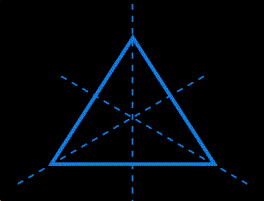Some other patterns also have three lines of symmetry.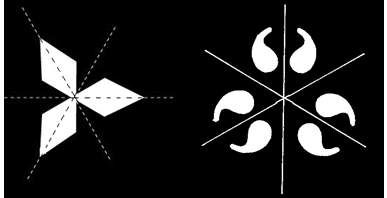### Four Lines of Symmetry

A square is symmetrical along four lines of symmetry, two along the diagonals and two along with the midpoints of the opposite sides.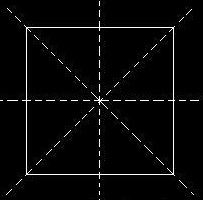Some other patterns also have four lines of symmetry.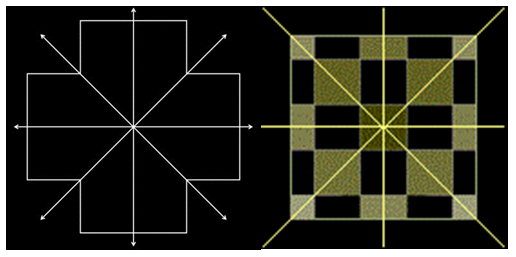### Five Lines of Symmetry

A regular pentagon has around five lines of symmetry. The lines joining a vertex to the mid-point of the opposite side divides the figure into ten symmetrical halves.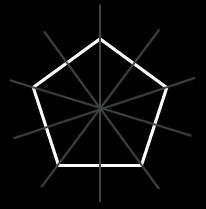Some other patterns also have five lines of symmetry, such as a star.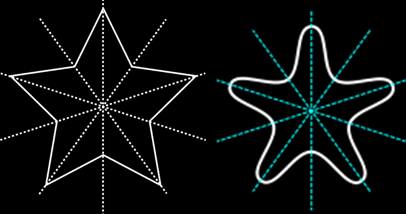### Six Lines of Symmetry

A regular hexagon is said to have six lines of symmetry, 3 joining the opposite vertices and 3 joining the mid-points of the opposite sides.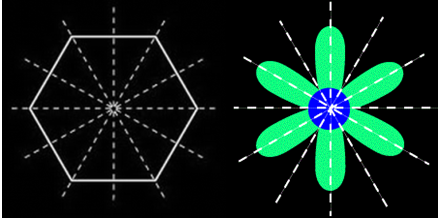Similarly, a regular polygon with N sides has N lines of symmetry.

### Infinite Lines of Symmetry

A circle has infinite or no lines of symmetry. It is symmetrical along all its diameters.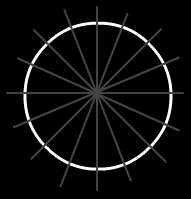### Line of Symmetry Examples

Some of the examples of the line of symmetry for different figures are given below-

1. A Triangle is said to have 3, 1 or even no lines of symmetry
2. A Quadrilateral has 4 or 2 or no lines of symmetry
3. Equilateral Triangle is said to have 3- lines of symmetry
4. Regular Pentagon has 5 lines of symmetry
5. A Regular Heptagon has 7 lines of symmetry